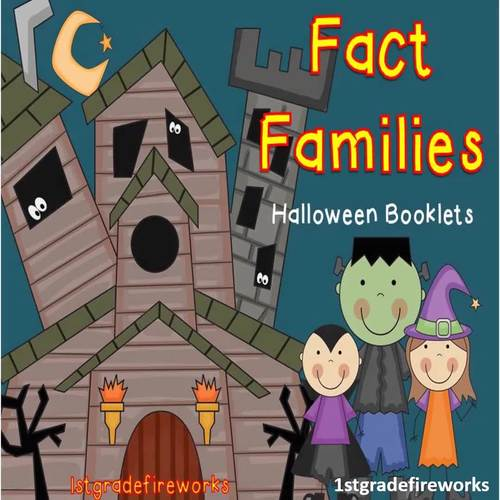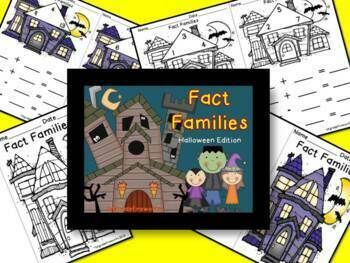# Math Addition & Subtraction Fact Families - Halloween Booklets

Rated 4.8 out of 5, based on 134 reviews
134 Ratings;
1st - 2nd
Subjects
Resource Type
Standards
Formats Included
• PDF
Pages
16 pages
\$4.00
List Price:
\$6.00
You Save:
\$2.00
\$4.00
List Price:
\$6.00
You Save:
\$2.00
Report this resource to TPT

#### What educators are saying

One of the best purchases I have made to support my teaching and students' independent practice of fact families.
My students loved these! They couldn't wait to color the cute pictures and the content really helped drive home the concept of fact families!

### Description

Are you teaching addition and subtraction fact families to align with number bonds and math mountains? Students LOVE making BOOKLETS! Easy Prep Fact Family Booklets in color and Black/White ( to save ink ). Students can color after they complete the page. WIN! WIN!

• Halloween-themed math practice booklets for addition and subtraction fact families.
• First Grade math number bonds for guided math centers, partner math stations, or independent math activities.
• Includes: cover, guided practice pages, and independent activity pages.
• Fact Family Booklet worksheets for whole group teaching, guided math for small groups, and/or independent math worksheet practice.
• Halloween-themed for easy prep and high student engagement.
• Available in Color & B/W.
Total Pages
16 pages
N/A
Teaching Duration
N/A
Report this resource to TPT
Reported resources will be reviewed by our team. Report this resource to let us know if this resource violates TPT’s content guidelines.

### Standards

to see state-specific standards (only available in the US).
Apply properties of operations as strategies to add and subtract. If 8 + 3 = 11 is known, then 3 + 8 = 11 is also known. (Commutative property of addition.) To add 2 + 6 + 4, the second two numbers can be added to make a ten, so 2 + 6 + 4 = 2 + 10 = 12. (Associative property of addition.)
Understand subtraction as an unknown-addend problem. For example, subtract 10 – 8 by finding the number that makes 10 when added to 8.
Relate counting to addition and subtraction (e.g., by counting on 2 to add 2).
Add and subtract within 20, demonstrating fluency for addition and subtraction within 10. Use strategies such as counting on; making ten (e.g., 8 + 6 = 8 + 2 + 4 = 10 + 4 = 14); decomposing a number leading to a ten (e.g., 13 - 4 = 13 - 3 - 1 = 10 - 1 = 9); using the relationship between addition and subtraction (e.g., knowing that 8 + 4 = 12, one knows 12 - 8 = 4); and creating equivalent but easier or known sums (e.g., adding 6 + 7 by creating the known equivalent 6 + 6 + 1 = 12 + 1 = 13).
Understand the meaning of the equal sign, and determine if equations involving addition and subtraction are true or false. For example, which of the following equations are true and which are false? 6 = 6, 7 = 8 - 1, 5 + 2 = 2 + 5, 4 + 1 = 5 + 2.